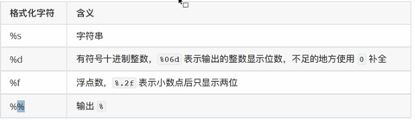# 1 加法器python中

``````##加法器
#输入第两个int类型的数字用于加法
a = input('请输入第一个数:')
#转成in了下
a = int(a)
b = input('请输入第二个数:')
b = int(b)
#相加
c = a + b
c = int(c)
#输出
print('结果是:%d'  %c)

``````

# 2 计算三位数各个位相加

``````# 计算三位数的各个位相加
##输入一个三位数
a = input('请输入一个三位数:')

# 分出来三个数
b = a

c = a

d = a

# 相加(转成数值型)
sum1 = int(b) + int(c) + int(d)

# 输出结果
print('%s + %s + %s = %d' % (b, c, d, sum1))
``````

# 3 计算三角形面积

``````##计算三角形面积

# 已知三边海伦公式得出三角形面积
##需要平方根所以引入数学公式
import math

# 输入三边
a = float(input('请输入a:'))

b = float(input('请输入b:'))

c = float(input('请输入c:'))

# 先求p，p = （a+b+c） / 2

p = (a + b + c) / 2

# 求s面积

s = math.sqrt(p * (p - a) * (p - b) * (p - c))

##输入面积

print("面积为：%.2f" % s)
``````

# 4 计算话费

if a <= xx ：a = 1

``````##要求假设话费1毛一分钟，
##需要向上取整函数倒入数学函数
import math
##通过输入的秒数计算话费
# 输入秒数
a = int( input("请输入通话秒数:"))
# 如果小于60s就不要钱
if a < 60: a = 1
## 不足一分钟按一分钟
else: a = math.ceil(a/60)
print("%d 毛" %a)

``````Python Free

QQ：417803890